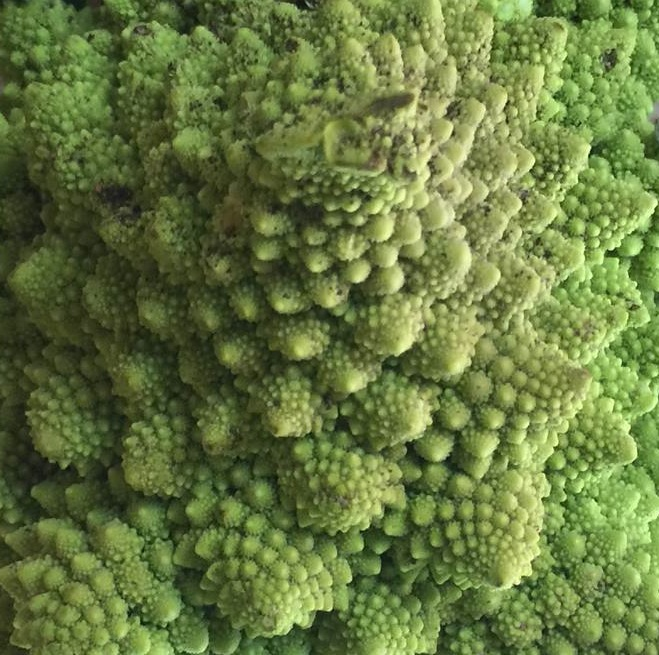In mathematics, a function is kind of the same thing as the function of an object, but not really. A mathematical function describes the relation between a set of inputs  and a set of outputs. Each input is related to exactly one output. We use mathematical functions all the time, ‘times two’ is a function: […]